# Effect of atmospheric drag on artificial satellite orbits

An artificial satellite that is in a low-altitude orbit around the Earth interacts with the upper atmosphere. Although the air in the upper atmosphere has a very low mass density, when integrated over a sufficiently long period of time, this interaction can significantly modify the satellite orbit, causing it to decay (i.e., to decrease in altitude). Let us investigate this effect. We shall work in the geocentric frame of reference introduced in the previous section.

The force per unit mass that the atmosphere exerts on an orbiting satellite can be modeled as (Batchelor 2000)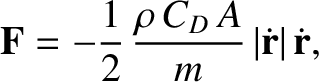(10.130)

where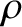is the mass density of the atmosphere (at the satellite's position),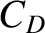the drag coefficient,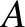the cross-sectional area of the satellite perpendicular to its direction of motion,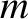the satellite mass, and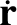the satellite velocity. It can be seen that the force is proportional to the square of the satellite speed, and is oppositely directed to its instantaneous direction of motion (i.e., the force constitutes a drag). The drag coefficient is an order unity dimensionless constant that depends primarily on the satellite's shape. For the case of a spherical satellite,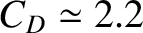(Cook 1965).

The density distribution of the terrestrial atmosphere is conveniently modeled as (de Pater and Lissauer 2010)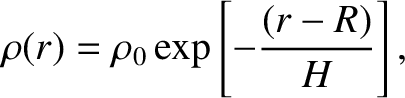(10.131)

where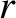measures radial distance from the Earth's center,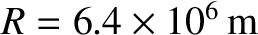is the terrestrial radius,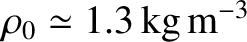the atmospheric density at ground level (i.e.,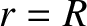), and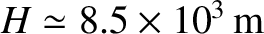the atmospheric scale height. Obviously, the previous formula is only valid when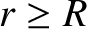.

Assuming that the atmospheric drag force, (10.130), is small compared to the force of gravitational attraction between the Earth and the satellite—and can, thus, be treated as a perturbation—the satellite's orbit can be modeled as Keplerian ellipse whose six elements evolve slowly in time under the influence of the drag. Letbe cylindrical coordinates in a reference frame that is aligned with the satellite's instantaneous orbital plane, as described in Section I.1. Here,is the satellite's true anomaly. (See Section 4.11.) Making use of the analysis of Appendix I, we can write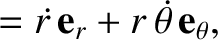(10.132)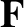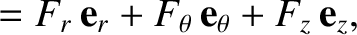(10.133)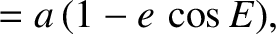(10.134)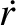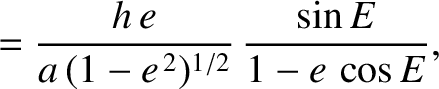(10.135)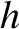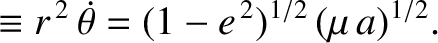(10.136)

Here,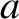,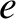,, andare the satellite's orbital major radius, eccentricity, angular momentum per unit mass, and eccentric anomaly, respectively. (See Section 4.11.) Moreover,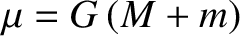, whereis the terrestrial mass. It follows from the previous equations that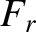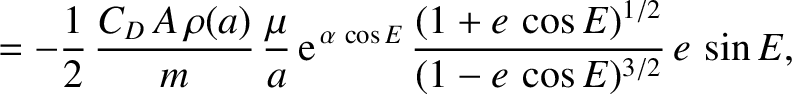(10.137)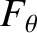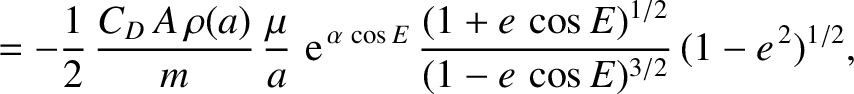(10.138)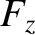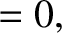(10.139)

where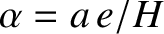.

As has already been mentioned, the satellite's instantaneous orbit is a Keplerian ellipse characterized by six orbital elements, which we choose to be the major radius,, the mean anomaly at epoch,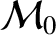, the eccentricity,, the argument of the perigee,, the inclination (to the Earth's equatorial plane),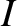, and the longitude of the ascending node (measured with respect to the vernal equinox),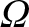. (See Section 4.12.) These elements evolve slowly in time, under the influence of the atmospheric drag force. For the case in hand, this time evolution is most conveniently specified in terms of the Gauss planetary equations, which take the form (see Appendix I)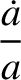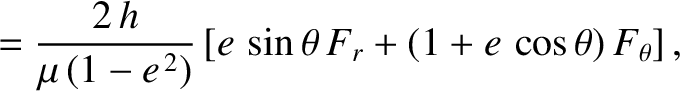(10.140)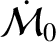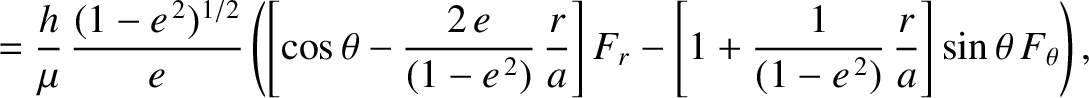(10.141)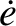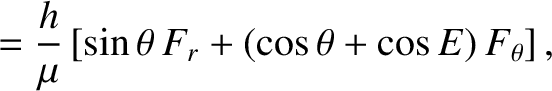(10.142)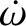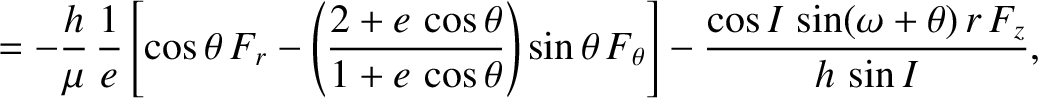(10.143)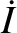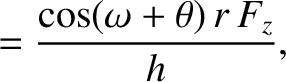(10.144)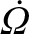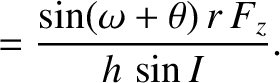(10.145)

Given that (see Section 4.11 and Exercise 16)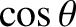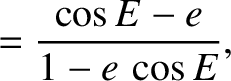(10.146)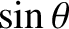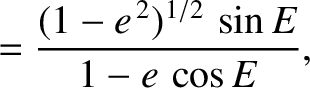(10.147)

the Gauss planetary equations can be combined with Equations (10.137)–(10.139) to give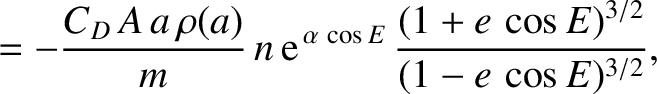(10.148)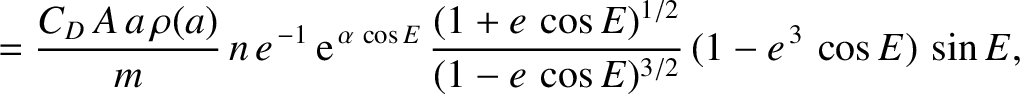(10.149)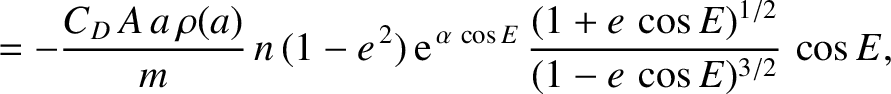(10.150)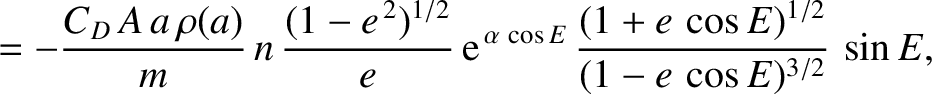(10.151)(10.152)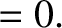(10.153)

Here,is the unperturbed mean orbital angular velocity. It is immediately apparent that the atmospheric drag force does not give rise to any change in the inclination of the satellite's orbital plane (becauseandare both constant). This is not surprising, because the drag force has no component normal to this plane (i.e.,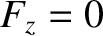).

As explained previously, we are working in the limit in which the atmospheric drag force is perturbative. This limit requires the relative changes in the satellite's orbital elements induced by the drag in an orbital period,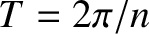, to all be small. It is apparent from Equations (10.148)–(10.151) that this is the case provided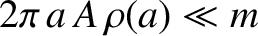; in other words, as long as the mass of air encountered by the satellite in a single orbit, which is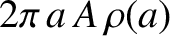, is much less than the satellite's mass. Assuming that we are in the perturbative limit (i.e.,), the evolution of the satellite's orbital elements takes place on a timescale that is much longer than its orbital period. We can concentrate on this evolution, and filter out any relatively short-term oscillations in the elements, by averaging expressions (10.148)–(10.151) over an orbital period. A suitable orbit-average operator is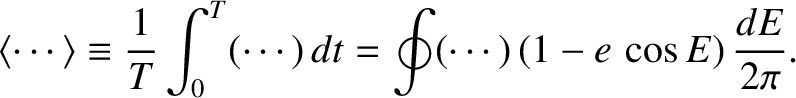(10.154)

Here, we have made use of the fact that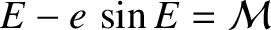, and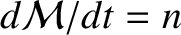, for a Keplerian orbit. (See Section 4.11.) Thus, we deduce that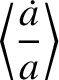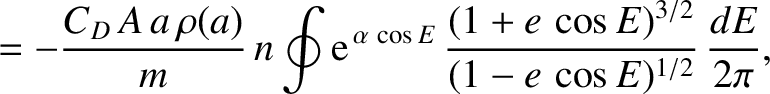(10.155)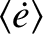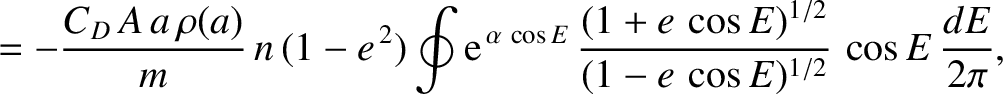(10.156)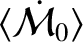(10.157)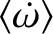(10.158)

It follows that the atmospheric drag force causes the major radius and eccentricity of the satellite's orbit to both decay monotonically in time [because the right-hand sides of Equations (10.155) and (10.156) are both negative]. On the other hand, the force does not produce any precession in the perigee of the orbit (because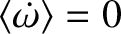). Furthermore, the fact that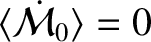implies that the drag force does not modify the Keplerian result that the mean orbital angular velocity is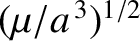. Now, it is easily demonstrated (see Exercise 4) that the satellite's orbit-averaged kinetic energy is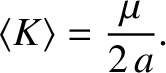(10.159)

Hence, we deduce that, despite the negative work done on the satellite by the atmospheric drag force, its kinetic energy actually increases as its orbit decays (i.e., asdecreases). Evidently, the negative work done on the satellite by the drag force is more than offset by the positive work done by gravity as its altitude decreases.